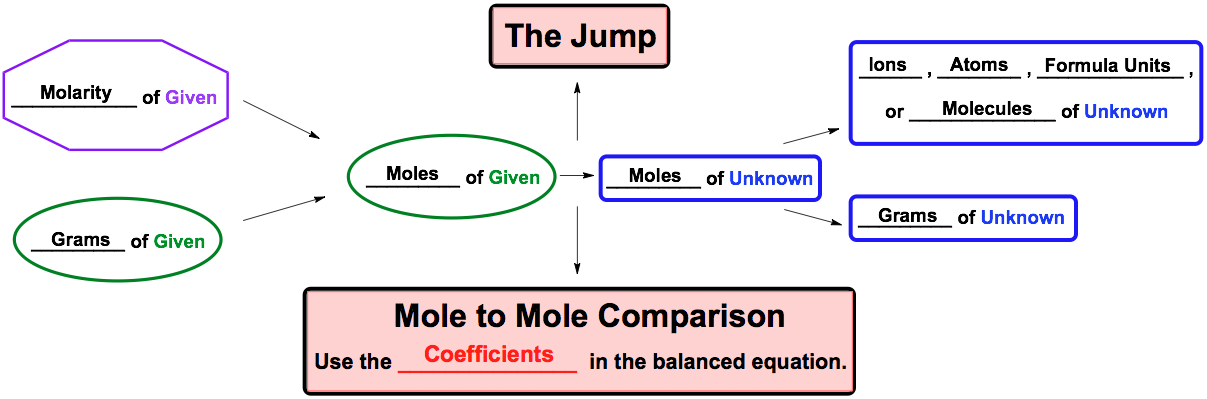Clutch Prep is now a part of Pearson
Ch.4 - Chemical Quantities & Aqueous ReactionsWorksheetSee all chapters

# Solution Stoichiometry

See all sections
Sections
Solutions
Molarity
Osmolarity
Dilutions
Solubility Rules
Electrolytes
Molecular Equations
Gas Evolution Equations
Solution Stoichiometry
Complete Ionic Equations
Calculate Oxidation Numbers
Redox Reactions
Balancing Redox Reactions: Acidic Solutions
Balancing Redox Reactions: Basic Solutions
Activity Series

Solution Stoichiometry deals with stoichiometric calculations in solutions that involve volume and molarity.

###### Solution Stoichiometry

Concept #1: Solution Stoichiometry

Use the solution stoichiometric chart when dealing with calculations involving molarity and/or volume.Example #1: How many moles of hydrogen gas were produced when 38.74 mL of 0.275 M H2O reacts with excess sodium?

Practice: How many milliliters of 0.325 M HCl are needed to react with 16.2 g of magnesium metal?

2 HCl (aq) + Mg (s) → MgCl2 + H2 (g)

Practice: What is the molar concentration of a hydrobromic acid solution if it takes 34.12 mL of HBr to completely neutralize 82.56 mL of 0.156 M Ca(OH)­2

2 HBr (aq) + Ca(OH)2 (aq)  → CaBr2 (aq) + 2 H2O (l)

Practice: Consider the following balanced chemical equation:

H2O+ 2 MnO4 + 3 SO32-  → 2 MnO2 + 3 SO42-+ 2 OH

How many grams of MnO2 (MW: 86.94 g/mol) will be created when 25.0 mL of 0.120 M MnO4 (MW: 118.90 g/mol) reacts with 32.0 mL of 0.140 M SO32- (MW: 80.07 g/mol).